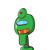# 10 or two third of the number of Apples in a box are eaten by Sam is Sam has three such boxes how many apples is he left with now​

10 or two third of the number of Apples in a box are eaten by Sam is Sam has three such boxes how many apples is he left with now​

### 1 thought on “10 or two third of the number of Apples in a box are eaten by Sam is Sam has three such boxes how many apples is he left with now​”

1.## 35

Step-by-step explanation:

10 = 2/3

Total number of boxes = 3

If, 2/3 = 10

Therefore, 1 = 10 × 3/2 ( Reciprocal of 2/3 )

1 box contains 15 apples

15 × 3 = 45

45 – 10 ( the apples he ate ) = 35 ans